# Function of exponential type

(diff) ← Older revision | Latest revision (diff) | Newer revision → (diff)

An entire functionsatisfying the condition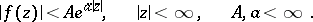Ifis represented by a series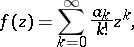thenThe simplest examples of functions of exponential type are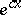,,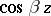, and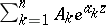.

A function of exponential type has an integral representation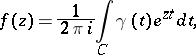where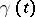is the function associated within the sense of Borel (see Borel transform) andis a closed contour enclosing all the singularities of.

How to Cite This Entry:
Function of exponential type. Encyclopedia of Mathematics. URL: http://encyclopediaofmath.org/index.php?title=Function_of_exponential_type&oldid=12134
This article was adapted from an original article by A.F. Leont'ev (originator), which appeared in Encyclopedia of Mathematics - ISBN 1402006098. See original article